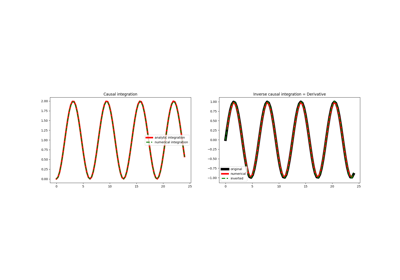# pylops.CausalIntegration¶

class pylops.CausalIntegration(N, dims=None, dir=-1, sampling=1, halfcurrent=True, dtype='float64')[source]

Causal integration.

Apply causal integration to a multi-dimensional array along dir axis.

Parameters: N : int Number of samples in model. dims : list, optional Number of samples for each dimension (None if only one dimension is available) dir : int, optional Direction along which smoothing is applied. sampling : float, optional Sampling step dx. halfcurrent : float, optional Add half of current value (True) or the entire value (False) dtype : str, optional Type of elements in input array.

Notes

The CausalIntegration operator applies a causal integration to any chosen direction of a multi-dimensional array.

For simplicity, given a one dimensional array, the causal integration is:

$y(t) = \int x(t) dt$

which can be discretised as :

$y[i] = \sum_{j=0}^i x[j] dt$

or

$y[i] = (\sum_{j=0}^{i-1} x[j] + 0.5x[i]) dt$

where $$dt$$ is the sampling interval. In our implementation, the choice to add $$x[i]$$ or just $$0.5x[i]$$ is made by selecting the halfcurrent parameter.

Note that the integral of a signal has no unique solution, as any constant $$c$$ can be added to $$y$$, for example if $$x(t)=t^2$$ the resulting integration is:

$y(t) = \int t^2 dt = \frac{t^3}{3} + c$

If we apply a first derivative to $$y$$ we in fact obtain:

$x(t) = \frac{dy}{dt} = t^2$

no matter the choice of $$c$$.

Attributes: shape : tuple Operator shape explicit : bool Operator contains a matrix that can be solved explicitly (True) or not (False)

Methods

 __init__(self, N[, dims, dir, sampling, …]) Initialize this LinearOperator. adjoint(self) Hermitian adjoint. cond(self, \*\*kwargs_eig) Condition number of linear operator. conj(self) Complex conjugate operator div(self, y[, niter]) Solve the linear problem $$\mathbf{y}=\mathbf{A}\mathbf{x}$$. dot(self, x) Matrix-matrix or matrix-vector multiplication. eigs(self[, neigs, symmetric, niter]) Most significant eigenvalues of linear operator. matmat(self, X) Matrix-matrix multiplication. matvec(self, x) Matrix-vector multiplication. rmatvec(self, x) Adjoint matrix-vector multiplication. transpose(self) Transpose this linear operator.

## Examples using pylops.CausalIntegration¶Causal Integration